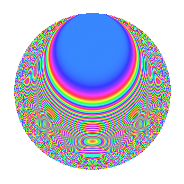# Properties

 Label 4009.2.a.cLevel 4009 Weight 2 Character orbit 4009.a Self dual Yes Analytic conductor 32.012 Analytic rank 1 Dimension 71 CM No

# Learn more about

## Newspace parameters

 Level: $$N$$ = $$4009 = 19 \cdot 211$$ Weight: $$k$$ = $$2$$ Character orbit: $$[\chi]$$ = 4009.a (trivial)

## Newform invariants

 Self dual: Yes Analytic conductor: $$32.0120261703$$ Analytic rank: $$1$$ Dimension: $$71$$ Fricke sign: $$1$$ Sato-Tate group: $\mathrm{SU}(2)$

## $q$-expansion

The dimension is sufficiently large that we do not compute an algebraic $$q$$-expansion, but we have computed the trace expansion.

 $$\operatorname{Tr}(f)(q) =$$ $$71q$$ $$\mathstrut -\mathstrut 15q^{2}$$ $$\mathstrut -\mathstrut 8q^{3}$$ $$\mathstrut +\mathstrut 69q^{4}$$ $$\mathstrut -\mathstrut 18q^{5}$$ $$\mathstrut -\mathstrut 9q^{6}$$ $$\mathstrut -\mathstrut 19q^{7}$$ $$\mathstrut -\mathstrut 39q^{8}$$ $$\mathstrut +\mathstrut 63q^{9}$$ $$\mathstrut +\mathstrut O(q^{10})$$ $$\operatorname{Tr}(f)(q) =$$ $$71q$$ $$\mathstrut -\mathstrut 15q^{2}$$ $$\mathstrut -\mathstrut 8q^{3}$$ $$\mathstrut +\mathstrut 69q^{4}$$ $$\mathstrut -\mathstrut 18q^{5}$$ $$\mathstrut -\mathstrut 9q^{6}$$ $$\mathstrut -\mathstrut 19q^{7}$$ $$\mathstrut -\mathstrut 39q^{8}$$ $$\mathstrut +\mathstrut 63q^{9}$$ $$\mathstrut -\mathstrut 10q^{10}$$ $$\mathstrut -\mathstrut 52q^{11}$$ $$\mathstrut -\mathstrut 9q^{12}$$ $$\mathstrut -\mathstrut 15q^{13}$$ $$\mathstrut -\mathstrut 53q^{14}$$ $$\mathstrut -\mathstrut 33q^{15}$$ $$\mathstrut +\mathstrut 53q^{16}$$ $$\mathstrut -\mathstrut 10q^{17}$$ $$\mathstrut -\mathstrut 35q^{18}$$ $$\mathstrut +\mathstrut 71q^{19}$$ $$\mathstrut -\mathstrut 33q^{20}$$ $$\mathstrut -\mathstrut 38q^{21}$$ $$\mathstrut -\mathstrut 6q^{22}$$ $$\mathstrut -\mathstrut 65q^{23}$$ $$\mathstrut -\mathstrut 30q^{24}$$ $$\mathstrut +\mathstrut 51q^{25}$$ $$\mathstrut -\mathstrut 4q^{26}$$ $$\mathstrut -\mathstrut 23q^{27}$$ $$\mathstrut -\mathstrut 29q^{28}$$ $$\mathstrut -\mathstrut 97q^{29}$$ $$\mathstrut -\mathstrut 27q^{30}$$ $$\mathstrut -\mathstrut 53q^{31}$$ $$\mathstrut -\mathstrut 78q^{32}$$ $$\mathstrut -\mathstrut 17q^{33}$$ $$\mathstrut -\mathstrut 24q^{34}$$ $$\mathstrut -\mathstrut 38q^{35}$$ $$\mathstrut +\mathstrut 24q^{36}$$ $$\mathstrut -\mathstrut 33q^{37}$$ $$\mathstrut -\mathstrut 15q^{38}$$ $$\mathstrut -\mathstrut 86q^{39}$$ $$\mathstrut +\mathstrut 25q^{40}$$ $$\mathstrut -\mathstrut 69q^{41}$$ $$\mathstrut +\mathstrut 64q^{42}$$ $$\mathstrut -\mathstrut 10q^{43}$$ $$\mathstrut -\mathstrut 94q^{44}$$ $$\mathstrut -\mathstrut 34q^{45}$$ $$\mathstrut -\mathstrut 6q^{46}$$ $$\mathstrut -\mathstrut 37q^{47}$$ $$\mathstrut -\mathstrut q^{48}$$ $$\mathstrut +\mathstrut 74q^{49}$$ $$\mathstrut -\mathstrut 41q^{50}$$ $$\mathstrut -\mathstrut 46q^{51}$$ $$\mathstrut -\mathstrut 30q^{52}$$ $$\mathstrut -\mathstrut 50q^{53}$$ $$\mathstrut -\mathstrut 17q^{54}$$ $$\mathstrut -\mathstrut 30q^{55}$$ $$\mathstrut -\mathstrut 116q^{56}$$ $$\mathstrut -\mathstrut 8q^{57}$$ $$\mathstrut +\mathstrut 11q^{58}$$ $$\mathstrut -\mathstrut 93q^{59}$$ $$\mathstrut -\mathstrut 56q^{60}$$ $$\mathstrut -\mathstrut 18q^{61}$$ $$\mathstrut -\mathstrut q^{62}$$ $$\mathstrut -\mathstrut 84q^{63}$$ $$\mathstrut +\mathstrut 93q^{64}$$ $$\mathstrut -\mathstrut 78q^{65}$$ $$\mathstrut -\mathstrut 53q^{66}$$ $$\mathstrut -\mathstrut 5q^{67}$$ $$\mathstrut -\mathstrut 9q^{68}$$ $$\mathstrut -\mathstrut 69q^{69}$$ $$\mathstrut -\mathstrut 10q^{70}$$ $$\mathstrut -\mathstrut 221q^{71}$$ $$\mathstrut -\mathstrut 73q^{72}$$ $$\mathstrut -\mathstrut 34q^{73}$$ $$\mathstrut -\mathstrut 58q^{74}$$ $$\mathstrut -\mathstrut 70q^{75}$$ $$\mathstrut +\mathstrut 69q^{76}$$ $$\mathstrut -\mathstrut 2q^{77}$$ $$\mathstrut +\mathstrut 7q^{78}$$ $$\mathstrut -\mathstrut 68q^{79}$$ $$\mathstrut -\mathstrut 71q^{80}$$ $$\mathstrut +\mathstrut 39q^{81}$$ $$\mathstrut +\mathstrut 26q^{82}$$ $$\mathstrut -\mathstrut 45q^{83}$$ $$\mathstrut -\mathstrut 10q^{84}$$ $$\mathstrut -\mathstrut 44q^{85}$$ $$\mathstrut -\mathstrut 80q^{86}$$ $$\mathstrut -\mathstrut 7q^{87}$$ $$\mathstrut -\mathstrut 46q^{88}$$ $$\mathstrut -\mathstrut 143q^{89}$$ $$\mathstrut +\mathstrut 41q^{90}$$ $$\mathstrut -\mathstrut 30q^{91}$$ $$\mathstrut -\mathstrut 46q^{92}$$ $$\mathstrut +\mathstrut 32q^{93}$$ $$\mathstrut +\mathstrut 41q^{94}$$ $$\mathstrut -\mathstrut 18q^{95}$$ $$\mathstrut -\mathstrut 140q^{96}$$ $$\mathstrut -\mathstrut 18q^{97}$$ $$\mathstrut -\mathstrut 97q^{98}$$ $$\mathstrut -\mathstrut 142q^{99}$$ $$\mathstrut +\mathstrut O(q^{100})$$

## Embeddings

For each embedding $$\iota_m$$ of the coefficient field, the values $$\iota_m(a_n)$$ are shown below.

For more information on an embedded modular form you can click on its label.

Label $$a_{2}$$ $$a_{3}$$ $$a_{4}$$ $$a_{5}$$ $$a_{6}$$ $$a_{7}$$ $$a_{8}$$ $$a_{9}$$ $$a_{10}$$
1.1 −2.82251 2.93474 5.96657 0.420997 −8.28333 −3.44749 −11.1957 5.61269 −1.18827
1.2 −2.70369 1.93926 5.30992 1.68888 −5.24315 4.83108 −8.94900 0.760725 −4.56619
1.3 −2.69837 1.88273 5.28118 −2.05530 −5.08030 0.784756 −8.85381 0.544674 5.54594
1.4 −2.67657 0.00971531 5.16401 0.0508514 −0.0260037 0.312050 −8.46867 −2.99991 −0.136107
1.5 −2.66661 −1.96401 5.11081 −2.76483 5.23725 2.50280 −8.29531 0.857341 7.37272
1.6 −2.61964 −2.82043 4.86252 −3.49446 7.38851 2.54132 −7.49878 4.95481 9.15424
1.7 −2.51184 −2.51408 4.30932 3.86977 6.31496 2.24136 −5.80064 3.32060 −9.72023
1.8 −2.48400 0.221625 4.17025 −2.50863 −0.550515 2.33062 −5.39089 −2.95088 6.23143
1.9 −2.35519 0.815593 3.54692 1.05865 −1.92088 −4.06376 −3.64328 −2.33481 −2.49333
1.10 −2.30773 −2.77619 3.32561 2.46750 6.40670 −0.00709873 −3.05915 4.70724 −5.69433
1.11 −2.28791 −0.433636 3.23453 1.92947 0.992121 3.12765 −2.82450 −2.81196 −4.41445
1.12 −2.25185 −1.69642 3.07082 −1.12007 3.82008 −4.02650 −2.41131 −0.122162 2.52222
1.13 −2.08913 2.53881 2.36446 0.545075 −5.30390 0.502260 −0.761409 3.44555 −1.13873
1.14 −2.08320 2.50442 2.33972 −4.26568 −5.21720 −3.89477 −0.707698 3.27211 8.88625
1.15 −1.98460 0.183292 1.93862 −1.97615 −0.363761 −5.05020 0.121816 −2.96640 3.92186
1.16 −1.93894 −1.61421 1.75950 −0.710068 3.12986 −1.51648 0.466323 −0.394323 1.37678
1.17 −1.89616 2.32800 1.59541 −3.08512 −4.41426 2.27635 0.767159 2.41961 5.84987
1.18 −1.89239 −0.431235 1.58115 3.97235 0.816065 −2.66937 0.792636 −2.81404 −7.51724
1.19 −1.74061 −3.34117 1.02972 −2.66559 5.81567 0.0455688 1.68888 8.16342 4.63975
1.20 −1.69413 −0.649307 0.870061 −0.0469641 1.10001 3.97959 1.91426 −2.57840 0.0795630
See all 71 embeddings
 $$n$$: e.g. 2-40 or 990-1000 Embeddings: e.g. 1-3 or 1.71 Significant digits: Format: Complex embeddings Normalized embeddings Satake parameters Satake angles

## Inner twists

This newform does not have CM; other inner twists have not been computed.

## Atkin-Lehner signs

$$p$$ Sign
$$19$$ $$-1$$
$$211$$ $$-1$$

## Hecke kernels

This newform can be constructed as the kernel of the linear operator $$T_{2}^{71} + \cdots$$ acting on $$S_{2}^{\mathrm{new}}(\Gamma_0(4009))$$.